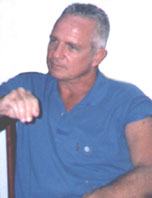On why cold fusion is denied by nuclear theorists Date: Friday, October 29, 2021 @ 11:28:33 GMTTopic: ScienceSuch subject must be considered from two viewpoints: 1- Why nuclear theorists reject the possibility of the existence of cold fusion? The answer is simple: because from the standard Coulomb’s law F= KQq/d² there is need to consider the existence of the strong nuclear force, so that to explain the stability of atomic nuclei. And from the assumption that strong nuclear force really promotes the stability of atomic nuclei, then theoretically cold fusion is impossible to occur. But experiments show that cold fusion exists. Then the second question is: 2- How can cold fusion be possible, as by considering the standard Coulomb’s law it is impossible? The  answer is again simple: because the standard Coulomb’s law is incomplete. Coulomb’s repulsions (and attraction) does not follow the equation F= KQq/d² for distances shorter than Bohr’s radius. For distances shorter than Bohr’s radius, Coulomb’s law is F= KQq/d^(X+Y), where X decreases with the decrease of the distance between the charges “Q” and “q”, and Y grows with relative speed between the charges.In my book “Subtle is the Math” the equation F= KQq/d^(X+Y) is proven by calculations, in the first paper of the book, entitled “Relation between QED, Coulomb’s Law and fine-structure constant”.  And why does Coulomb’s repulsions vary with the parameter Y in the equation F= KQq/d^(X+Y) ?This happens because, as shown in that paper, the line-forces of the electric fields are composed by particles (captured from the quantum vacuum) that move with the speed of light. The interaction of the fields of two charges Q and q depends on their relative velocity. The faster is their relative velocity, than stronger is the interaction between the particles that compose the fields of the charges Q and q. Therefore:1- If two charges Q and q are moving against each other with relativistic speed V, the electric interaction between Q and q varies proportional to V+c, since the particles of the quantum vacuum (that compose the electric fields of Q and q) are moving with velocity “c”.2- If the charge Q is at rest, and the charge q is moving away the charge Q with relativistic speed V, the electric interaction between Q and q varies proportional to c-V.  This explains why, in the U238 alpha-decay, despite the potential energy of the alpha particle is 27 MeV when it leaves the U238, in the experiments it is detected with only 4 MeV. As shown in my book “Subtle is the Math”, the Gamow’s hypothesis of quantum tunneling is not able to explain this paradox.In the stars, the relative velocity between particles as protons, deuterons, etc., plays a fundamental role in the process of nuclear synthesis, because the repulsion between two protons moving against each other grows with the growth of their relative speeds, and that’s why, despite the strong nuclear force does not exist, in the stars the fusion between two protons is very hard. Otherwise, if the velocity did not play a fundamental role, the Sun of our planetary system would waste its hydrogen in some few minutes.Perhaps we may say that such property, of the growth of Coulomb’s repulsions with the growth of the relative velocity between two charged particles, represents a strong force. It is a special type of strong force, with depends on the speed of the two charges.  But it does not exist for two charged particles (with low speed), inside atomic nuclei.From this new Coulomb’ law there is no need to consider the strong nuclear force.  Several puzzles of nuclear physics are solved by considering that strong force does not exist. For instance, it explains why two neutrons do not form a dineutron, whereas by considering the strong force two neutrons would have to fuse and form a dineutron, since there is not repulsion between them, but they have a strong attraction by the strong nuclear force.  The Heisenberg’s proposal of isospin does not solve the puzzle.In resume, from a new nuclear physics, in which the strong force does not exist, the cold fusion becomes possible.Therefore, acceptation of the existence of cold fusion depends on the acceptation that standard Coulomb’s law is incomplete.This question, as to why nuclear physicists reject cold fusion, is beyond the understanding of most people interested in cold fusion issues. For example, yesterday I submitted an ad here on ZPEnergy, communicating the publication of my book Subtle is the Math, by St Honorť. And Editor Vlad didn't run my ad in "Home" because he mistakenly assumes that my book has nothing to do with the subject of cold fusion. [vlad: it was posted here]But actually, as my book is proposing new laws for the foundations of physics, its content concerns all fields of physics.Wladimir_Guglinski